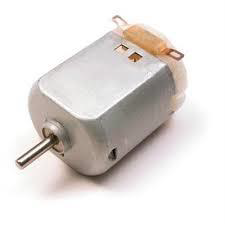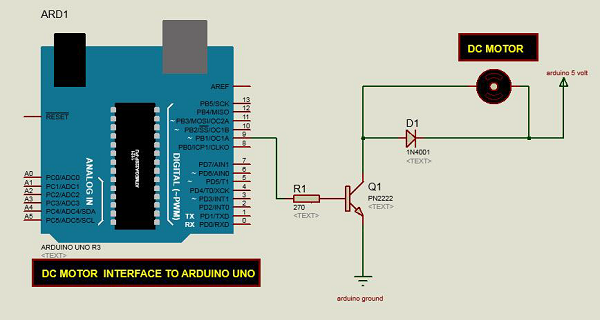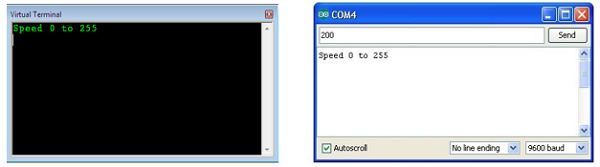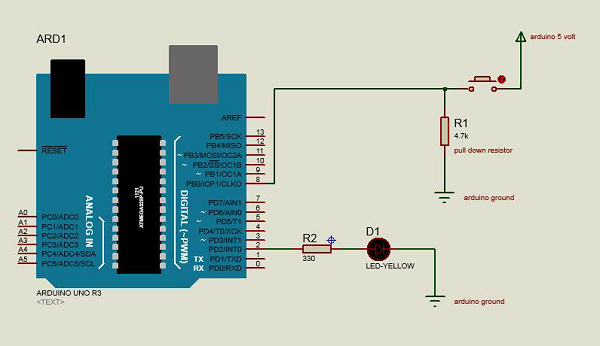# Arduino - 直流电机

• 直流电机
• 伺服电机
• 步进电机• 只需让你的电机旋转
• 控制电机速度
• 控制直流电机的旋转方向

### 需要的组件

• 1x Arduino UNOArduino 板
• 1x PN2222 晶体管
• 1x 小型 6V 直流电机
• 1x 1N4001 二极管
• 1x270Ω 电阻

### 程序### 注意事项

• 首先，确保晶体管以正确的方式连接。如图所示，晶体管的扁平侧面应朝向 Arduino 板。

• 其次，根据图像中所示的布置，二极管的条纹端应朝向+5V 电源线。

### Spin ControlArduino Code

int motorPin = 3;

void setup() {

}

void loop() {
digitalWrite(motorPin, HIGH);
}


## 电机速度控制### Arduino 代码

int motorPin = 9;

void setup() {
pinMode(motorPin, OUTPUT);
Serial.begin(9600);
while (! Serial);
Serial.println("Speed 0 to 255");
}

void loop() {
if (Serial.available()) {
int speed = Serial.parseInt();
if (speed >= 0 && speed <= 255) {
analogWrite(motorPin, speed);
}
}
}


### 代码注意loop 函数中，命令 Serial.parseInt 用于读取在串行监视器中作为文本输入的数字，并将其转换为 int。你可以在这里输入任何数字。如果数字在 0 到 255 之间，则下一行中的 if 语句只使用此数字进行模拟写入。

## 旋转方向控制### 需要的组件

• 1×L298 桥接 IC
• 1×直流电机
• 1×Arduino UNO
• 1×面包板
• 10×跳线

### 程序IN1 IN2 电机行为

1 向前转
1 向后转
1 1 制动

IC L298 的引脚 IN1 连接到 Arduino 的引脚 8，而 IN2 连接到引脚 9。Arduino 的这两个数字引脚控制电机的方向。IC 的 EN A 引脚连接到 Arduino 的 PWM 引脚 2。这将控制电机的速度。

### 连接步骤

• 将 5V 和 IC 的地线分别连接到 5V 和 Arduino 的地。
• 将电机连接到 IC 的引脚 2 和 3。
• 将 IC 的 IN1 连接到 Arduino 的引脚 8。
• 将 IC 的 IN2 连接到 Arduino 的引脚 9。
• 将 IC 的 EN1 连接到 Arduino 的引脚 2。
• 将 IC 的 SENS 引脚连接到地。
• 使用 Arduino USB 线连接 Arduino，并使用 Arduino IDE 软件将程序上传到 Arduino。
• 使用电源，电池或 USB 电缆为 Arduino 板供电。

### Arduino 代码

const int pwm = 2 ; //initializing pin 2 as pwm
const int in_1 = 8 ;
const int in_2 = 9 ;
//For providing logic to L298 IC to choose the direction of the DC motor

void setup() {
pinMode(pwm,OUTPUT) ; //we have to set PWM pin as output
pinMode(in_1,OUTPUT) ; //Logic pins are also set as output
pinMode(in_2,OUTPUT) ;
}

void loop() {
//For Clock wise motion , in_1 = High , in_2 = Low
digitalWrite(in_1,HIGH) ;
digitalWrite(in_2,LOW) ;
analogWrite(pwm,255) ;
/* setting pwm of the motor to 255 we can change the speed of rotation
by changing pwm input but we are only using arduino so we are using highest
value to driver the motor */
//Clockwise for 3 secs
delay(3000) ;
//For brake
digitalWrite(in_1,HIGH) ;
digitalWrite(in_2,HIGH) ;
delay(1000) ;
//For Anti Clock-wise motion - IN_1 = LOW , IN_2 = HIGH
digitalWrite(in_1,LOW) ;
digitalWrite(in_2,HIGH) ;
delay(3000) ;
//For brake
digitalWrite(in_1,HIGH) ;
digitalWrite(in_2,HIGH) ;
delay(1000) ;
}